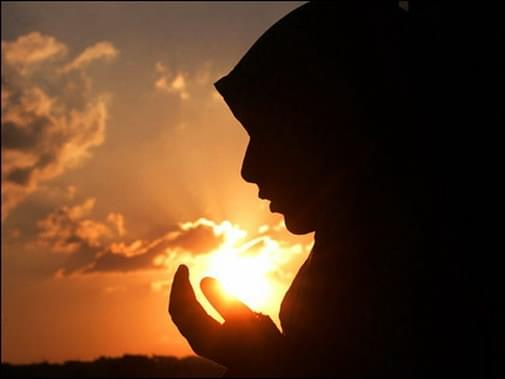General Poems

# A poem about the Muslim Woman.What do you see
when you look at me
Do you see someone limited,
or someone free

<=><=><=><=><=><=><=><=>

All some people can do is just look and stare
Simply because they can’t see my hair

<=><=><=><=><=><=><=><=>

Others think I am controlled and uneducated
They think that I am limited and un-liberated

<=><=><=><=><=><=><=><=>

They are so thankful that they are not me
Because they would like to remain ‘free’

<=><=><=><=><=><=><=><=>

Well free isn’t exactly the word I would’ve used
Describing women who are cheated on and abused

<=><=><=><=><=><=><=><=>

They think that I do not have opinions or voice
They think that being hooded isn’t my choice

<=><=><=><=><=><=><=><=>

They think that the hood makes me look caged
That my husband or dad are totally outraged

<=><=><=><=><=><=><=><=>

All they can do is look at me in fear
And in my eye there is a tear

<=><=><=><=><=><=><=><=>

Not because I have been stared at or made fun of
But because people are ignoring the one up above

<=><=><=><=><=><=><=><=>

On the day of judgment they will be the fools
Because they were too ashamed to play by their own rules

<=><=><=><=><=><=><=><=>

Maybe the guys won’t think I am a cutie
But at least I am filled with more inner beauty

<=><=><=><=><=><=><=><=>

See I have declined from being a guy’s toy
Because I won’t let myself be controlled by a boy

<=><=><=><=><=><=><=><=>

Real men are able to appreciate my mind
And aren’t busy looking at my behind

<=><=><=><=><=><=><=><=>

Hooded girls are the ones really helping the muslim cause
The role that we play definitely deserves applause

<=><=><=><=><=><=><=><=>

I will be recognized because I am smart and bright
And because some people are inspired by my sight

<=><=><=><=><=><=><=><=>

The smart ones are attracted by my tranquility
In the back of their mind they wish they were me

<=><=><=><=><=><=><=><=>

We have the strength to do what we think is right
Even if it means putting up a life long fight

<=><=><=><=><=><=><=><=>

You see we are not controlled by a mini skirt and tight shirt
We are given only respect, and never treated like dirt

<=><=><=><=><=><=><=><=>

So you see, we are the ones that are free and liberated
We are not the ones that are sexually terrorized and violated

<=><=><=><=><=><=><=><=>

We are the ones that are free and pure
We’re free of STD’s that have no cure

<=><=><=><=><=><=><=><=>